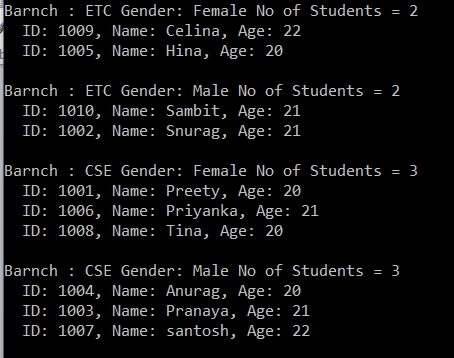# Linq GroupBy Method with Multiple Keys

## Linq GroupBy Method with Multiple Keys

In this article, I am going to discuss the Linq GroupBy Method with Multiple Keys in C# with some examples. Please read our previous article before proceeding to this article where we discussed the Linq GroupBy Method in C# with examples in C#. As part of this article, we will discuss the following pointers.

1. Why do we need to group the data based on multiple keys?
2. How to use the Linq GroupBy Method with Multiple Keys in C#?
3. Examples using both Method and Query Syntax.
##### Why do we need to group the data based on Multiple keys?

In real-time applications, we need to group the data based on multiple keys. So in this article, we will see how to group the data based on multiple keys. But before that, you just need to remember one thing that when you are using multiple keys in Group By operator then the data returned is an anonymous type.

##### Student Helper Class:

We are going to use the following Student class. Please create a class with the name Student and then copy and paste the following code in it.

```using System.Collections.Generic;
namespace GroupByDemo
{
public class Student
{
public int ID { get; set; }
public string Name { get; set; }
public string Gender { get; set; }
public string Barnch { get; set; }
public int Age { get; set; }

public static List<Student> GetStudents()
{
return new List<Student>()
{
new Student { ID = 1001, Name = "Preety", Gender = "Female",
Barnch = "CSE", Age = 20 },
new Student { ID = 1002, Name = "Snurag", Gender = "Male",
Barnch = "ETC", Age = 21  },
new Student { ID = 1003, Name = "Pranaya", Gender = "Male",
Barnch = "CSE", Age = 21  },
new Student { ID = 1004, Name = "Anurag", Gender = "Male",
Barnch = "CSE", Age = 20  },
new Student { ID = 1005, Name = "Hina", Gender = "Female",
Barnch = "ETC", Age = 20 },
new Student { ID = 1006, Name = "Priyanka", Gender = "Female",
Barnch = "CSE", Age = 21 },
new Student { ID = 1007, Name = "santosh", Gender = "Male",
Barnch = "CSE", Age = 22  },
new Student { ID = 1008, Name = "Tina", Gender = "Female",
Barnch = "CSE", Age = 20  },
new Student { ID = 1009, Name = "Celina", Gender = "Female",
Barnch = "ETC", Age = 22 },
new Student { ID = 1010, Name = "Sambit", Gender = "Male",
Barnch = "ETC", Age = 21 }
};
}
}
}```
##### Example:

The following example group the students first by Branch and then by Gender. The student groups first sorted by Branch in descending order and then by Gender in ascending order. Finally, the students in each group are sorted by their names in ascending order

```using System;
using System.Linq;
namespace GroupByDemo
{
class Program
{
static void Main(string[] args)
{
//Using Method Syntax
var GroupByMultipleKeysMS = Student.GetStudents()
.GroupBy(x => new { x.Barnch, x.Gender })
.OrderByDescending(g => g.Key.Barnch).ThenBy(g => g.Key.Gender)
.Select(g => new
{
Branch = g.Key.Barnch,
Gender = g.Key.Gender,
Students = g.OrderBy(x => x.Name)
});
//Using Query Syntax
var GroupByMultipleKeysQS = from student in Student.GetStudents()
group student by new
{
student.Barnch,
student.Gender
} into stdGroup
orderby stdGroup.Key.Barnch descending,
stdGroup.Key.Gender ascending
select new
{
Branch = stdGroup.Key.Barnch,
Gender = stdGroup.Key.Gender,
Students = stdGroup.OrderBy(x => x.Name)
};

//It will iterate through each group
foreach (var group in GroupByMultipleKeysQS)
{
Console.WriteLine(\$"Barnch : {group.Branch} Gender: {group.Gender} No of Students = {group.Students.Count()}");
//It will iterate through each item of a group
foreach (var student in group.Students)
{
Console.WriteLine(\$"  ID: {student.ID}, Name: {student.Name}, Age: {student.Age} ");
}
Console.WriteLine();
}
}
}
}

```

Output:In the next article, I am going to discuss the Linq ToLookup Operator in C# with some examples. Here, in this article, I try to explain how to use the Linq GroupBy Method with Multiple Keys with Examples. I hope you enjoy this article.

### 2 thoughts on “Linq GroupBy Method with Multiple Keys”

1.Implementation not given for above example

1.Thank you for letting us know. Implementation is provided. Kindly check now.